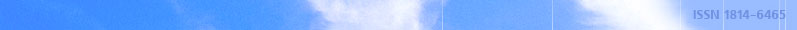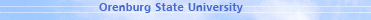december 07, 2021 RU/EN
 Headings of Vestnik Pedagogics Psychology Other

 Search Author Article

# 2006, № 9

Sokotushenko V.N. EQUATION OF SMALL MECHANICAL AGITATION IN TOUGH ISOTROPIC MEDIUM WITH ACCOUNTING OF THE SECOND COEFFICIENT OF TENACITYConsequences of equation of tough medium particles movement in contrast to elastic medium are not wave equation because of tenacity coefficient presence in it. Wave equations which satisfy functions of density, presser and the first variant of deformation speed tensor of ideally compressible medium particles follow at equal to zero tenacity coefficient from differential equation received in work which are constitute consequences of Navie – Stocks equation. Equations of small mechanical agitation in tough isotropic medium are presented with accounting of the second coefficient of tenacity.Editor-in-chief Sergey Aleksandrovich MIROSHNIKOV